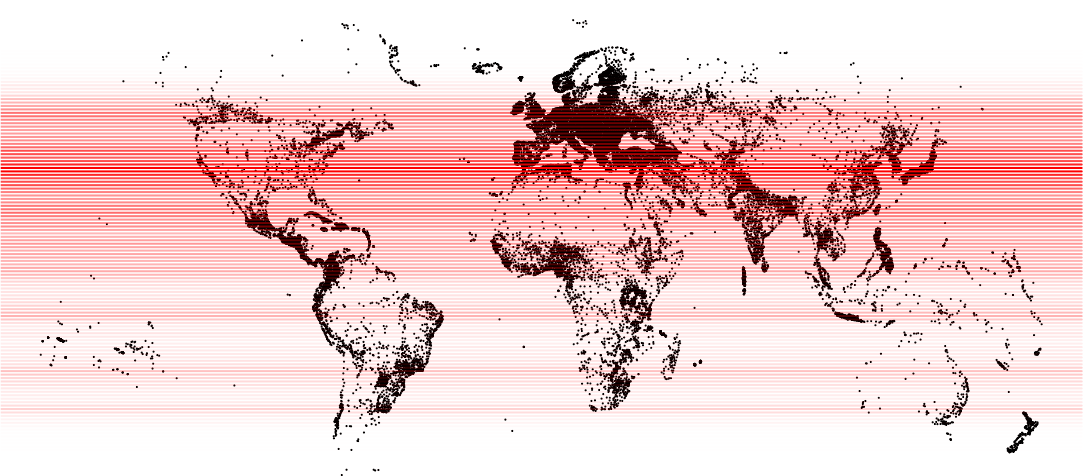Want to share your content on R-bloggers? click here if you have a blog, or here if you don't.

There was an interesting map on reddit this morning, with a visualisation of latitude and longituge of where people live, on Earth. So I tried to reproduce it. To compute the density, I used a kernel based approch

```> library(maps)
> data("world.cities")
> X=world.cities[,c("lat","pop")]
> liss=function(x,h){
+   w=dnorm(x-X[,"lat"],0,h)
+   sum(X[,"pop"]*w)
+ }
> vx=seq(-80,80)
> vy=Vectorize(function(x) liss(x,1))(vx)
> vy=vy/max(vy)
> plot(world.cities\$lon,world.cities\$lat,)
> for(i in 1:length(vx))
+ abline(h=vx[i],col=rgb(1,0,0,vy[i]),lwd=2.7)```For the other axis, we use a miror technique, to ensure that -180 is close the +180

```> Y=world.cities[,c("long","pop")]
> Ya=Y; Ya[,1]=Y[,1]-360
> Yb=Y; Yb[,1]=Y[,1]+360
> Y=rbind(Y,Ya,Yb)
> liss=function(y,h){
+   w=dnorm(y-Y[,"long"],0,h)
+   sum(Y[,"pop"]*w)
+ }
> vx=seq(-180,180)
> vy=Vectorize(function(x) liss(x,1))(vx)
> vy=vy/max(vy)
> plot(world.cities\$lon,world.cities\$lat,pch=19)
> for(i in 1:length(vx))
+ abline(v=vx[i],col=rgb(1,0,0,vy[i]),lwd=2.7)```Now we can add the two, on the same graph